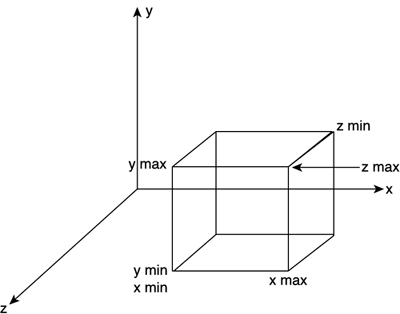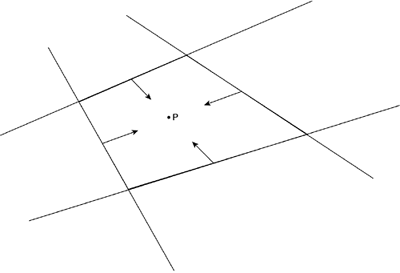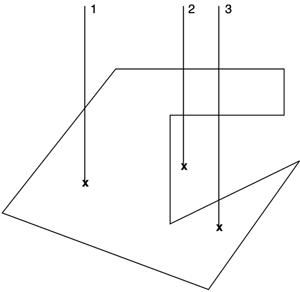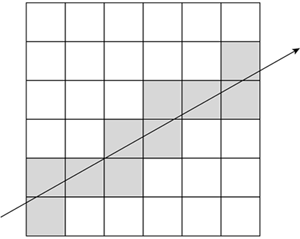# Point Inclusion Tests

The first problem we will try to analyze is finding whether a 2D or 3D point lies within different types of bodies, from spheres to meshes of various types. This is an essential test for fields like AI or collision detection. From grenades to wall-following, almost every interesting algorithm needs, at some point in time, to deal with this kind of issue.

#### Point-in-Sphere

The simplest point inclusion test involves testing whether a point is actually inside a sphere. A sphere can be considered a generalization of a point. It is actually a point with a radius. Thus, given the sphere (as shown in Figure 22.1)

` (X-Xc)2 + (Y-Yc)2 + (Z-Zc)2 = R2 `
##### Figure 22.1. Graphical representation of point-in-sphere test.and the point P

` P=(px,py,pz) `

P is inside the sphere if and only if

` Inside= (sqrt ( (px-xc)2 + (py-yc)2 + (pz-zc)2 ) < radius `

Remember that square roots are expensive. Thus, an optimization could be

` Inside= ( (px-xc)2 + (py-yc)2 + (pz-zc)2 ) < radius2 `

as well as storing the radius squared to speed up computations.

#### Point-in-AABB

An axis-aligned bounding box (AABB) is a box whose support planes are aligned with the X, Y, and Z planes (see Figure 22.2). It is very popular for gross collision detection. An object is assigned an AABB, and as a first step in the collision detection process, we test for collisions with the box, not the object, providing an almost trivial rejection case for most tests.

##### Figure 22.2. Graphical representation of an AABB.Thus an AABB is defined by six planes, whose equations are

` x-xmax=0 x+xmin=0 y-ymax=0 y+ymin=0 z-zmax=0 z+zmin=0 `

The test is as follows:

` bool inside(point p) { if (p.x>xmax) return false; if (p.x<xmin) return false; if (p.y>ymax) return false; if (p.y<ymin) return false; if (p.z>zmax) return false; if (p.z<zmin) return false; return true; } `

#### Point-in-Convex Polygon

We can compute whether a point is inside a convex polygon (see Figure 22.3) by looping through the edges of the polygon and making sure the point is always in the same hemispace with regard to the edges. We start at the first vertex and compute the vector from the first to the second. Then, we build a new vector from the first vertex to the point we are testing and perform a cross product between these two vectors. With the original vector and the result of the cross product, we can compute a plane that includes the edge. Then, we just need to test which hemispace from the plane the point located to. Repeating this for each edge of the polygon and making sure all hemispace tests return the same sign, we detect whether the point is inside the polygon.

##### Figure 22.3. Convex polygon with all normals pointing inward.Now, doing dot and cross products all over the place does not look very efficient. But all this can be precomputed. And after all, storing one plane per edge is just four floating-point values, which is a reasonable memory footprint. Here is the pseudocode in full. Notice how I assume normals to the polygon's faces are looking inward.

` While we haven't done a full cycle    Compute edge vector    Use edge vector and normal to compute "up" vector using a cross product    Use edge and up to compute a plane    If the plane value of point P is less than zero return false; End while Return true `

#### Point-in-Polygon (Convex and Concave): Jordan Curve Theorem

Computing whether a point is inside a polygon in a general case is significantly harder than performing the test when assuming the polygon is convex. One of the main techniques used to do this involves using the Jordan Curve Theorem, which states that a point is inside a 2D closed polygon if and only if the number of crossings from a ray emanating from the point in an arbitrary direction and the edges of the polygon is odd. Think of a triangle, for example. If a point is inside the triangle, and you create a ray from that point in any direction, it will cross the triangle at exactly one point.

The elegance of the Jordan Curve Theorem is that it only needs the polygon to be closed, because open polygons do not clearly specify the notion of "in" and "out." Notice, however, that the algorithm works beautifully on convex, concave, or even malformed polygons as shown in Figure 22.4.

##### Figure 22.4. Jordan Curve Theorem with several different cases analyzed.Thus, the algorithm can be described by the following pseudocode:

` bool isinside(point p, polygon P) choose an arbitrary direction... (1,0,0) is a good one build ray r based on p and the direction initialize count to zero for each edge       test ray-segment       if crossed             increase count       end if end for return count is odd `

So, the algorithm is O(n of edges), which is not too bad.

#### Point-in-Convex Object

Testing whether a point is inside a convex object is relatively straightforward and can be easily considered an extension of the point-in-convex shape test explained earlier. The 2D test works by checking the point against the line segments and making sure we are always in the same hemispace. In the 3D case, we will need to test for planes instead of line segments, but overall the approach is the same. A 3D point lies inside a 3D convex object if and only if the point is located in the same hemispace regarding the support planes of all the triangles of the object. Here is the algorithm in detail:

` sign=sign of the point-plane test using the first plane of support for each plane of support    if sign is different than the point-plane test sign for the next plane       return false end for return true `

Notice how we can perform early-bird detection because the test will return false as soon as one plane stops following the rule.

Point-in-convex object tests are very useful for collision detection. They can be efficiently performed on the convex hull of the objects we need to test. Their cost is O(number of planes), which can be greatly improved by adding a point versus bounding sphere test to discard most cases beforehand. For those cases returning a positive, we can choose between further refining the solution (if the object was actually concave) or using this simplified solution. Remember that the convex hull test can return a false positive for concave objects if the point lies in a cavity of the object. In this case, some games will require a higher precision result, and thus we will need to use an additional test to determine if there actually was a collision. A good option is to use the Jordan Curve 3D test, which is explained in the next section. But many games can use this simplified test based on convex objects with no problem at all.

Another option is to always decompose any concave objects into a set of convex objects, so this test can always be used safely. Although this strategy must be addressed carefully (sliding collisions do not work well in concave geometry), we can often avoid using a concave test, which will indeed be more costly.

If you need information on how to compute the convex hull of an object, check out the section "Computing a Convex Hull," at the end of this chapter, where a number of different strategies are presented.

#### Point-in-Object (Jordan Curve Theorem)

The 2D Jordan Curve Theorem explained earlier can be easily extended to 3D, thus obtaining a general point-object inclusion algorithm. The operation, again, would involve counting the intersections from the point along a ray in an arbitrary direction. If the number is odd, we are inside the object. If it is even, we are outside.

#### Point-in-Object (3DDDA)

The Jordan Curve method has a cost linear to the number of triangles, because we need to count intersections with a line segment. A different approach can greatly reduce this cost by increasing the memory footprint of the algorithm. It is called the 3D Digital Differential Analyzer (3DDDA). Its core idea is very simple. At load time, the process meshes, so data is stored in a 3D regular grid. Each grid cell will contain those triangles whose barycenter is located inside the cell. The grid size must be inversely proportional to the number of triangles in the mesh. So, when we need to find out whether a point is inside the mesh, all we have to do is follow the segment on a cell-by-cell basis, intersecting only with those triangles that lie in the cells we visit along the way. By doing so, we will end up testing a very low number of triangles, especially when compared to the whole mesh (see Figure 22.5).

##### Figure 22.5. 3DDDA, pictured. Visited cells are shaded.Another improvement to this algorithm is to store one enumerated value per cell, which can be INSIDE, OUTSIDE, and DON'T KNOW. As we load the mesh, we perform our 3DDDA test stochastically in several positions of each cell and store the result. This way, whenever we need to test for point inclusion, we can save most tests. If the grid size is selected properly, most cells will be completely in or out, and thus the test will be free. For those cells that have a part inside and a part outside, we can use our regular 3DDDA code.

3DDDA was introduced by Fujimoto as a way to speed up ray-mesh tests in ray tracing. Assuming N triangles and a grid of Gx,Gy,Gz cells, and assuming triangles are spaced evenly, the cost of a 3DDDA run is O(N/(Gx*Gy*Gz)) for a single cell, and at most we scan max (Gx,Gy,Gz) cells along the way. So, cost is orders of magnitude below the previous test. Accelerations in the hundredths are not uncommon, with the downside being the large memory footprint left by 3DDDA.Core Techniques and Algorithms in Game Programming2003
ISBN: N/A
EAN: N/A
Year: 2004
Pages: 261